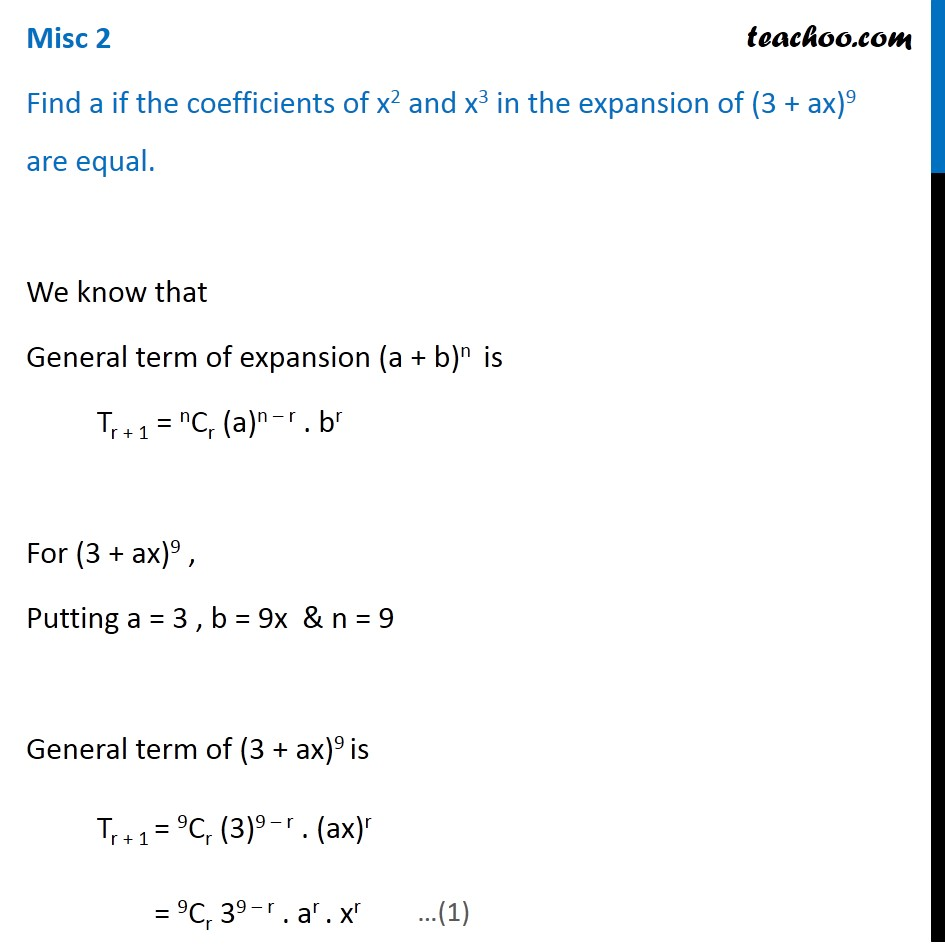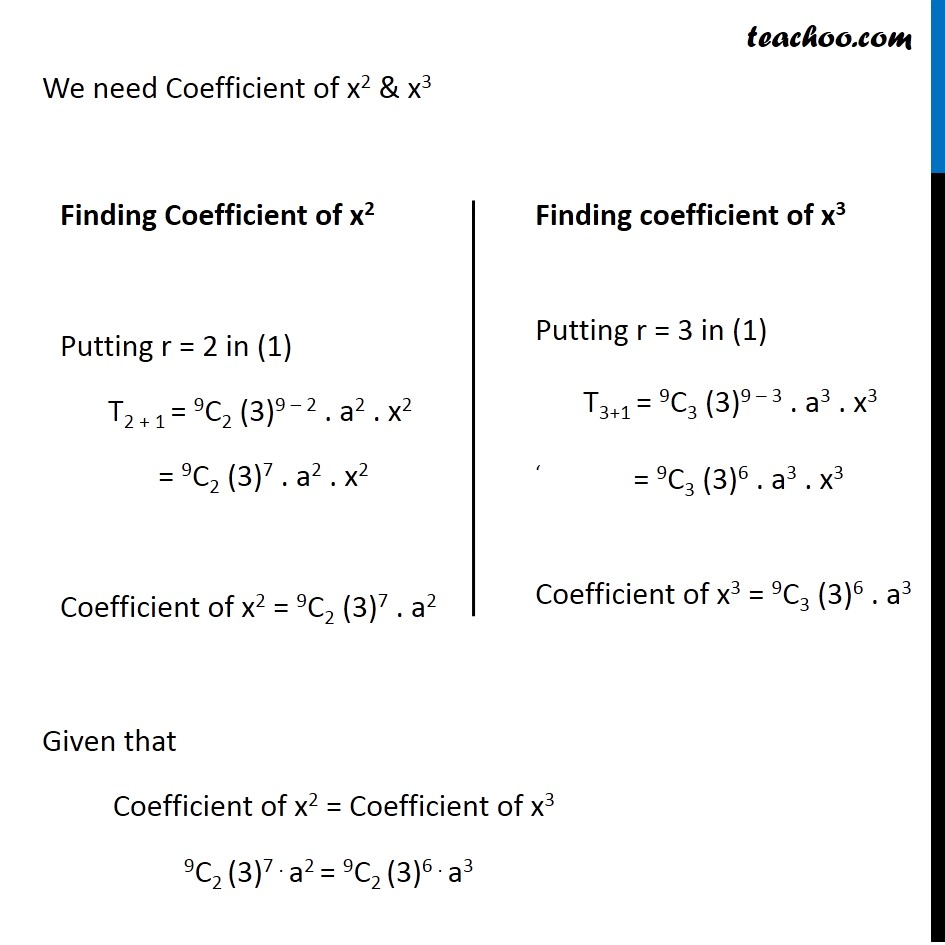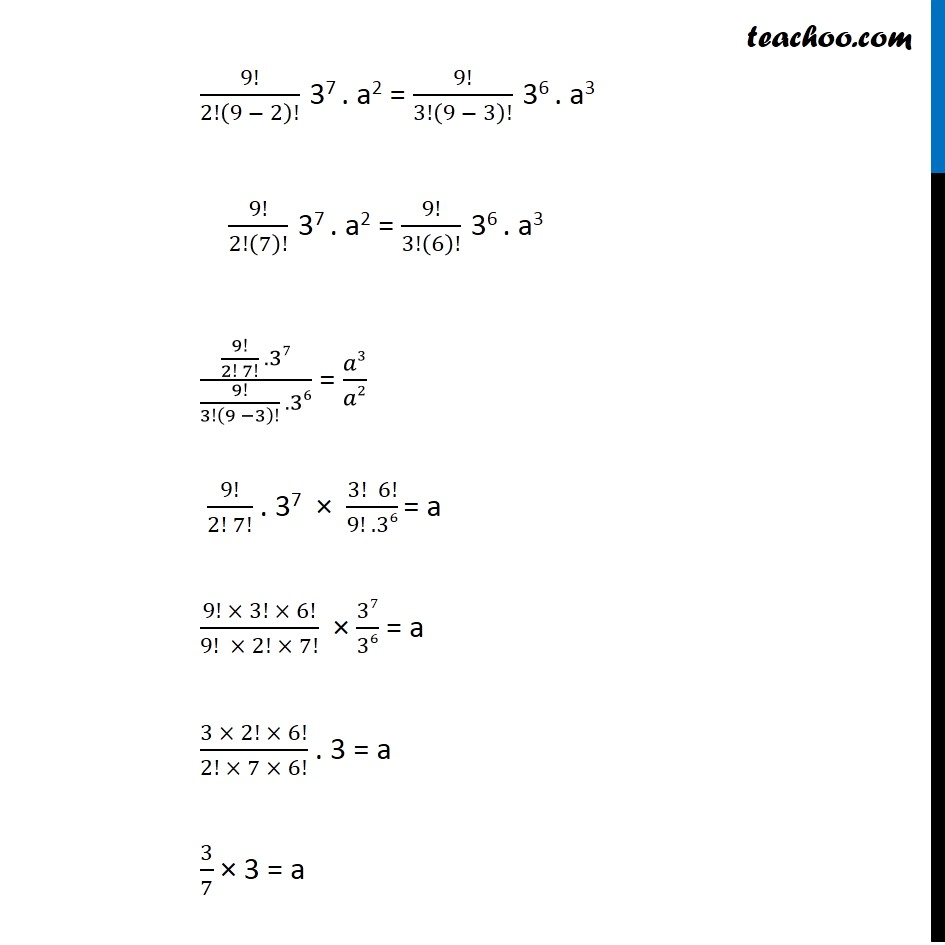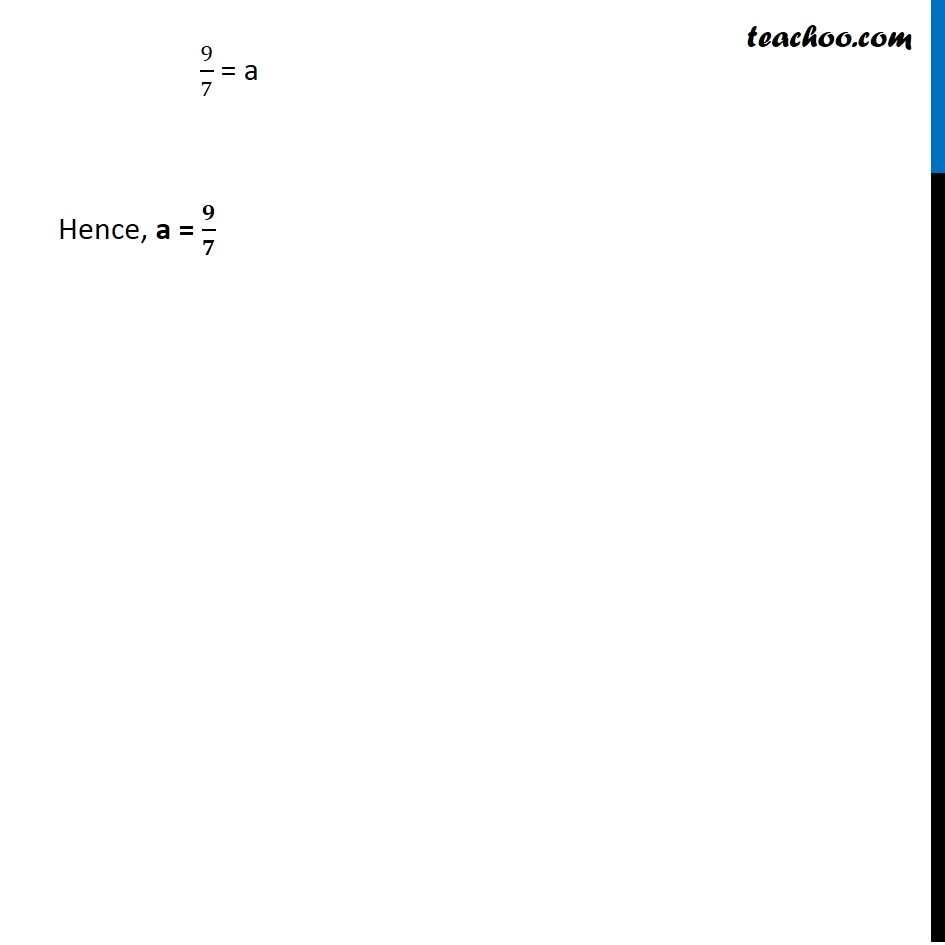Miscellaneous

Chapter 7 Class 11 Binomial Theorem
Serial order wiseLearn in your speed, with individual attention - Teachoo Maths 1-on-1 Class

### Transcript

Question 2 Find a if the coefficients of x2 and x3 in the expansion of (3 + ax)9 are equal. We know that General term of expansion (a + b)n is Tr + 1 = nCr (a)n – r . br For (3 + ax)9 , Putting a = 3 , b = 9x & n = 9 General term of (3 + ax)9 is Tr + 1 = 9Cr (3)9 – r . (ax)r = 9Cr 39 – r . ar . xr We need Coefficient of x2 & x3 Given that Coefficient of x2 = Coefficient of x3 9C2 (3)7 . a2 = 9C2 (3)6 . a3 Finding Coefficient of x2 Putting r = 2 in (1) T2 + 1 = 9C2 (3)9 – 2 . a2 . x2 = 9C2 (3)7 . a2 . x2 Coefficient of x2 = 9C2 (3)7 . a2 Finding coefficient of x3 Putting r = 3 in (1) T3+1 = 9C3 (3)9 – 3 . a3 . x3 ‘ = 9C3 (3)6 . a3 . x3 Coefficient of x3 = 9C3 (3)6 . a3 9!/2!(9 − 2)! 37 . a2 = 9!/3!(9 − 3)! 36 . a3 9!/2!(7)! 37 . a2 = 9!/3!(6)! 36 . a3 (9!/(2! 7!) . 37)/(9!/3!(9 −3)! . 36) = 𝑎3/𝑎2 9!/(2! 7!) . 37 × (3! 6!)/(9! . 36) = a (9! × 3! × 6!)/(9! × 2! × 7!) × 37/36 = a (3 × 2! × 6!)/(2! × 7 × 6!) . 3 = a 3/7 × 3 = a 9/7 = a Hence, a = 𝟗/𝟕